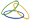##CADACUS, INC.

SOLUTIONS FOR SYSPRO™

As you are looking for Solutions to your SYSPRO™ needs, there may be other choices, but ask them if they have been working exclusively with SYSPRO™ since 1991. Ask them if they use the same NetExpress development system and tools SYSPRO uses. Very few other companies can say yes to both answers, but we can!

Providing quality solutions to the SYSPRO™ user community since 1991!

# Scripting for EDI - Multiple Insert Afters Multiple Times Method 2

#### Introduction

The script below is an example of how to perform multiple "Insert Afters" in a row multiple times.  Each function can only insert one segment.  Therefore the functions must be chained together to insert multiple segments.

This sample inserts two N1, N3 and N4 segments by having using the EDI.Value function to keep track of where in the process the functions are.  When set to 1, the first set of segments is inserted.  It is then set to 2 and the 2nd set inserted using the same functions.

```

' Script to do multiple insert afters and multiple N1, N3 and N4 segmets - Method two

Function XXX()   ' Replace XXX with the segment you need to insert the segments after

'  Setup to call the function "Insert_N1" to insert the first N1 segment

EDI.iValue("Pass") = 1  ' Variable is set to tell the functions this is pass 1
EDI.InsertAfterFunction = "Insert_N1"  ' Tell the system to call 'Insert_N1' instead of the default 'InsertAfter' function
EDI.InsertAfter = True
End Function

Function Insert_N1()
EDI.InsertSegment = "N1"

If EDI.iValue("Pass") = 1 Then
EDI.Element(1) = "Qualifier"
EDI.Element(2) = "Customer Name"
EDI.Element(3) = "Location Qualifier"
EDI.Element(4) = "Location Code"
Else
EDI.Element(1) = "2nd Qualifier"
EDI.Element(2) = "2nd Customer Name"
EDI.Element(3) = "2nd Location Qualifier"
EDI.Element(4) = "2nd Location Code"
End If

' After inserting the N1 segment, the following tells the system to insert another segment
' using the function "Insert_N3"

EDI.InsertAfterFunction = "Insert_N3"
EDI.InsertAfter = True
End Function

Function Insert_N3()
EDI.InsertSegment = "N3"

If EDI.iValue("Pass") = 1 Then
EDI.Element(1) = "Address Line 1"
EDI.Element(2) = "Address line 2"
Else
EDI.Element(1) = "2nd Address Line 1"
EDI.Element(2) = "2nd Address line 2"
End If

' After inserting the N3 segment, the following tells the system to insert another segment
' using the function "Insert_N4"

EDI.InsertAfterFunction = "Insert_N4"
EDI.InsertAfter = True
End Function

Function Insert_N4()
EDI.InsertSegment ="N4"

If EDI.iValue("Pass") = 1 Then
EDI.Element(1) = "City"
EDI.Element(2) = "State"
EDI.Element(3) = "Postal Code"
EDI.Element(4) = "Country Code"

' We want a 2nd set ot N1, N2, N3 segments. Change the "Pass" variable to 2 to indicate the
' 2nd pass and start again with the "Insert_N1" function.

EDI.iValue("Pass") = 2
EDI.InsertAfterFunction = "Insert_N1"
EDI.InsertAfter = True
Else
EDI.Element(1) = "2nd City"
EDI.Element(2) = "2nd State"
EDI.Element(3) = "2nd Postal Code"
EDI.Element(4) = "2nd Country Code"
End If
End Function
```

### Current Releases

SYSPRO™ :  April 4, 2021
Handheld :  April 4, 2021